﻿ 基于负载均衡的电力通信业务路径规划算法 Load Balance Based-Power Communication Service Path Programming Algorithm

Smart Grid
Vol. 08  No. 06 ( 2018 ), Article ID: 27509 , 9 pages
10.12677/SG.2018.86053

Load Balance Based-Power Communication Service Path Programming Algorithm

Tongqing Xu, Haoliang Zhang, Guofeng Liu, Haojun Zhao, Enze Zhang, Can Zhang

State Grid Jiangsu Electric Power Co., Ltd., Nanjing Power Supply Branch, Nanjing JiangsuReceived: Oct. 14th, 2018; accepted: Oct. 28th, 2018; published: Nov. 13th, 2018ABSTRACT

This paper designs a power communication service path planning algorithm, which effectively improves the balance of power communication service weight distribution, and reduces the risk of path fault to power communication service. This algorithm takes the balanced weight distribution of power communication service as goal. Firstly, in the nodes whose hops are less than threshold value, a feasible path is selected for service. Then, K path with the smallest distance as candidate path of business is chosen. Finally, the path with balanced distribution of service weights is selected as business allocation path from candidate path, so as to ensure balanced distribution of business weights.

Keywords:Electric Power Communication Service, Path Programming, Balance1. 引言

2. 问题模型

2.1. 问题描述

2.2. 问题处理流程

3. 基于负载均衡的电力通信业务路径规划算法

3.1. 路径规划算法

$BuildPath\left(G\left(N,E\right)\right)$ 从节点 ${n}_{i}\in N$ 的邻节点计算可行路径和候选路径。随后，从候选路径中选择业务权重分布均衡的路径作为业务部署路径，从而保障业务分布的均衡性。其中，业务权重由电力通信业务的安全级别决定，例如：继电保护业务权重为10，办公业务权重为1，A为已分配业务路径集合，B为备选路径集合，C为路径集合，S为备选节点集合， $\alpha$ 为跳数的门限值，例如16。 $InitPath\left(G\left(N,E\right)\right)$ 将与节点 ${n}_{i}$ 距离最小的节点放入业务路径集合A，其中，距离是 ${n}_{i}$ 每个邻节点上部署的业务权重。 $SelectNextNode\left({n}_{j}\right)$ 遍历 ${n}_{j}$ 的邻节点，从 ${n}_{j}$ 的可行路径中，选择k条业务权重最低的路径，作为备选路径。 $SetService\left({n}_{j},P\right)$ 从k个备选路径中，选择业务权重分布均衡的路径，作为业务分配路径，从而保障业务权重分布的均衡性。

$BuildPath\left(G\left(N,E\right)\right)$ 算法流程如图1所示：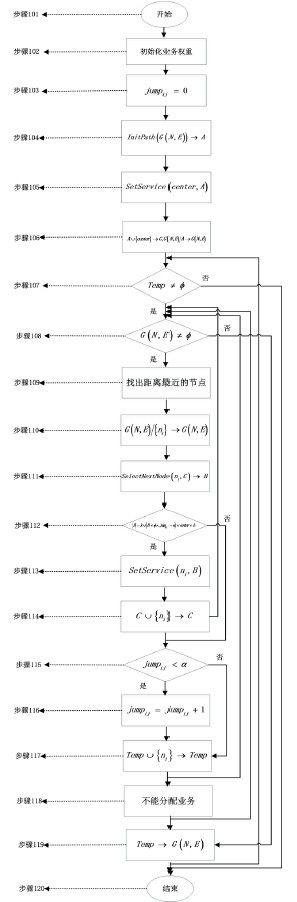Figure 1.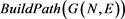algorithm flow chart

3.2. 可行路径选择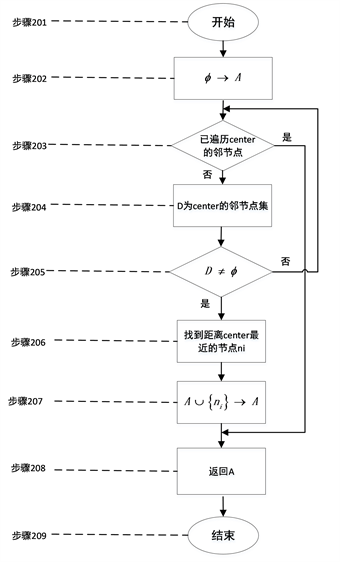Figure 2. $InitPath\left(G\left(N,E\right)\right)$ algorithm flow chart

$InitPath\left(G\left(N,E\right)\right)$ 算法流程如图2所示：

3.3. 备选路径选择

$SelectNextNode\left({n}_{j}\right)$ 算法流程如图3所示：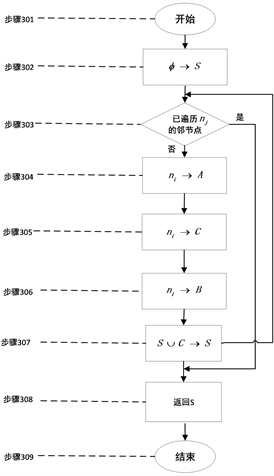Figure 3. $SelectNextNode\left({n}_{j}\right)$ algorithm flow chart

3.4. 业务路径筛选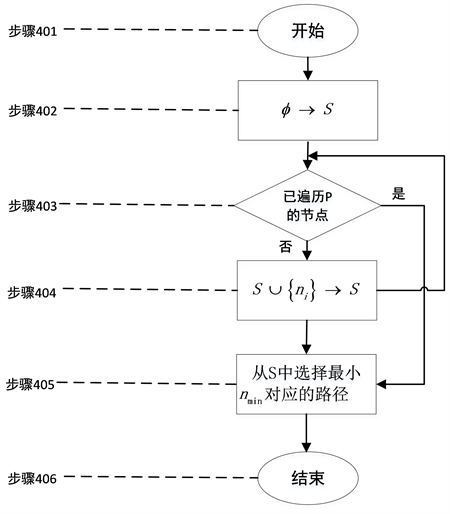Figure 4. $SetService\left({n}_{j},P\right)$ algorithm flow chart

$SetService\left({n}_{j},P\right)$ 算法流程如图4所示：

4. 实例分析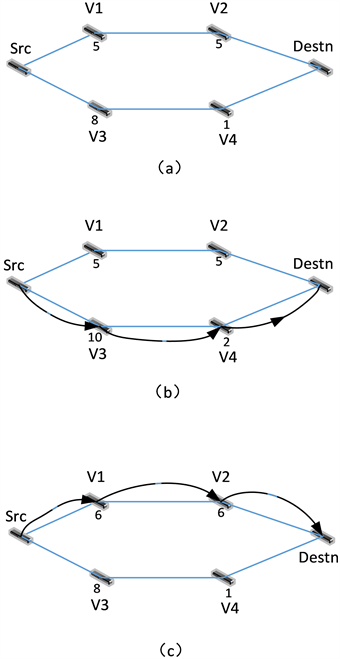Figure 5. Comparison of business deployment results

5. 结束语

Load Balance Based-Power Communication Service Path Programming Algorithm[J]. 智能电网, 2018, 08(06): 489-497. https://doi.org/10.12677/SG.2018.86053

1. 1. 吕顺利, 杨济海, 邓伟, 施健, 陆涛. Apriori-AHP算法在电力通信网业务风险评估中的研究及应用[J]. 计算机与数字工程, 2018, 46(4): 667-671.

2. 2. 吕玉祥, 杨阳, 稂龙亚, 王红全. 电力通信业务模型研究[J]. 自动化与仪器仪表, 2017(8): 180-182.

3. 3. 王勇, 利韶聪, 陈宝仁. 电力通信业务应用及发展分析[J]. 电力系统通信, 2010, 31(11): 44-47.

4. 4. 赵子岩, 张大伟. 国家电网公司“十二五”电力通信业务需求分析[J]. 电力系统通信, 2011(5): 56-60.

5. 5. 袁训明, 资浩. 分组传送网对于电力通信业务的传送性能研究[J]. 电力系统通信, 2012, 33(6): 58-62.

6. 6. 曾庆涛, 张国翊, 郭少勇, 邱雪松, 孟洛明. 面向可用性的电力通信业务通道路由选择算法[J]. 北京邮电大学学报, 2015, 38(6): 24-27.

7. 7. 郑蓉蓉, 赵子岩, 刘识, 庄自超. 基于重要度的电力通信业务路由分配算法[J]. 电力信息化, 2012, 10(10): 23-28.